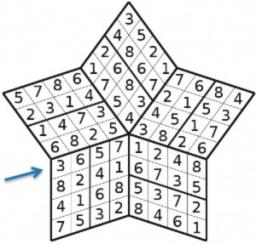# Difference 3101

The difference between the two numbers is 4/3. Specify both numbers if you know that the first number is 50% larger than the second number.

a =  4
b =  8/3 = 2 23

### Step-by-step explanation:Did you find an error or inaccuracy? Feel free to write us. Thank you!

Tips for related online calculators
Need help calculating sum, simplifying, or multiplying fractions? Try our fraction calculator.